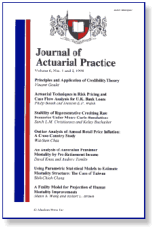Finance DepartmentJournal of Actuarial Practice 1993-2006

2000

Article

Citation

Journal of Actuarial Practice 8 (2000), pp. 237-256

Copyright 2000 Absalom Press

Abstract

This paper finds that when report lags are assumed to be independent, the age-to-age factor method produces biased estimates when applied to claim count development data. Two distributions are considered as models for the ultimate number of claims for an accident period: (0 a Poisson distribution, and (ii) a negative binomial distribution. In the Poisson case, the assumption of independent report lags implies the independence of the total number of claims reported in any two periods. In the negative binomial case, however, assuming that report lags are independent does not imply that increments are independent, and a somewhat different argument is required. Finally, it is proved that weighted average forecasts exhibit a smaller bias than do straight average estimates.

COinS